## Abstract

In this paper, we propose a novel penetration metric, called deformable penetration depth PDd, to define a measure of inter-penetration between two linearly deforming tetrahedra using the object norm. First of all, we show that a distance metric for a tetrahedron deforming between two configurations can be found in closed form based on object norm. Then, we show that the PDd between an intersecting pair of static and deforming tetrahedra can be found by solving a quadratic programming (QP) problem in terms of the distance metric with non-penetration constraints. We also show that the PDd between two, intersected, deforming tetrahedra can be found by solving a similar QP problem under some assumption on penetrating directions, and it can be also accelerated by an order of magnitude using pre-calculated penetration direction. We have implemented our algorithm on a standard PC platform using an off-the-shelf QP optimizer, and experimentally show that both the static/deformable and deformable/deformable tetrahedra cases can be solvable in from a few to tens of milliseconds. Finally, we demonstrate that our penetration metric is three-times smaller (or tighter) than the classical, rigid penetration depth metric in our experiments.

## Contributions

• In order to define PDd, we generalize the concept of object norm to a deformable case, and show that a distance metric for a tetrahedron deforming between two configurations can be found in closed form.

• Case 1: we show that the PDd between an intersected pair of one static and one deforming tetrahedra can be found by solving a quadratic programming (QP) problem over all possible penetrating directions in terms of the distance metric with non-penetration constraints.

• Case 2: we show that the PDd between an intersected pair of deforming tetrahedra can be found by solving a similar QP problem.

• Case 3: We approximate the case 2 using rigid penetration depth calculation based on the separating axis theorem, and demonstrate that this computation can be accelerated by an order of magnitude compared to the case 2 while the approximation error is kept to less than by 5%.

• We compare the metric results of our deformable penetration depth against those of rigid penetration depth and show that our results are three-times tighter than the rigid case.

## Metric Formulation

We propose a novel penetration metric, called deformable penetration depth PDd, to define a measure of inter-penetration between two linearly deforming tetrahedra using the object norm σ. Given an intersecting pair of linearly deforming tetrahedra, T1, T2, with the corresponding, initial configurations q0T1, q0T2 , their PDd is:

where C ∈ R24 is the contact (configuration) space imposed by T1, T2. Note that the proposed PDd is a generalization of the classical rigid (or translational) penetration depth.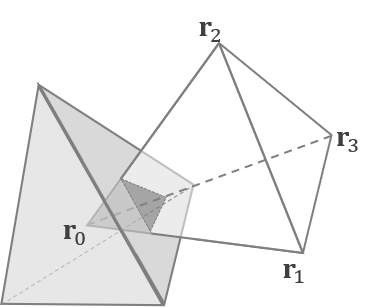##### (a) Rest State q0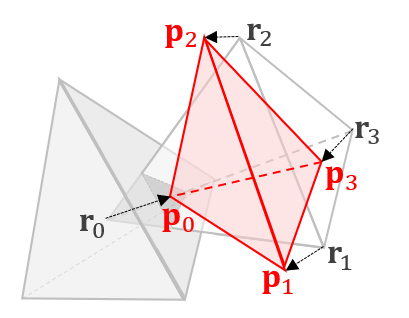##### (b) Deformed State q1

Fig. 1. Linear deformation of a tetrahedron to resolve inter-penetration from the rest q0 to deformed configuration q1.

## Results

As illustrated in Fig. 5, every result using PDd resolves penetration.The average performance results of each case are shown in Table 1. The running time for the static/deformable case is slightly faster than the deformable/deformable case since a fewer number of variables are required to optimize. The accelerated deformable/deformable case can be calculated in 1.07 milliseconds on average.

STAT/DEF DEF/DEF DEF/DEF(ACCEL.)
Performance 32.26 ms 46.29 ms 1.07 ms
PDd/PD 43.59% 27.94% 29.39%
##### Table 1. Performance statistics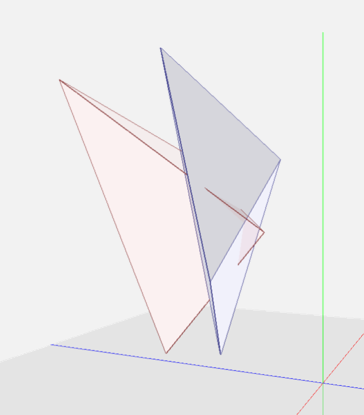##### (a) Penetrated State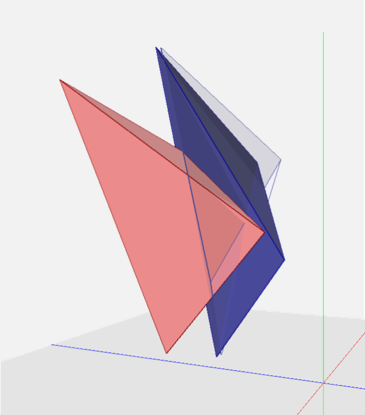##### (b) Static / Deformable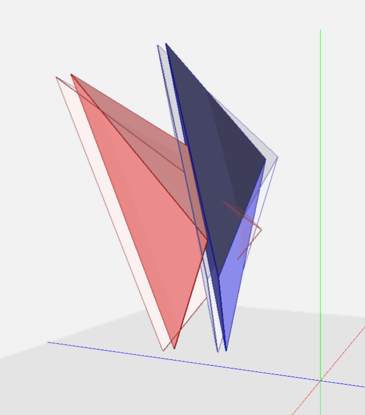##### (c) Deformable/ Deformable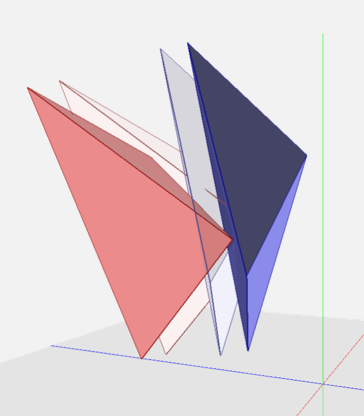##### (d) Rigid PD

Fig. 5. Implementation results of deformable penetration depth and rigid penetration depth. (a) shows the initial penetrated state of two tetrahedra. In (b)~(d), the solid colored tetrahedra are the results with PDd = 0.5692, 0.3469, PD = 1.496, respectively.

To the best of our knowledge, there are no penetration depth algorithms for deformable models available to guarantee full separation as a result (i.e. penetration metric with a tight upper bound). All the known penetration depth algorithms for deformable models provide only a lower bound, which does not guarantee full penetration resolution. Thus, it is unfair to compare our algorithms against existing algorithms for deformables and instead we compare our algorithms with rigid PD, which can be considered as an upper bound for deformable penetration depth.
In this case, in order to show the tightness of the metric upper bounds, we calculate the relative magnitude of PDd with respect to rigid penetration depth PD. Fig. 6 shows that the magnitude of PDd is less than 1 3 of that of the rigid case most times. This means that PDd provides a much tighter deformation metric than using the rigid penetration depth.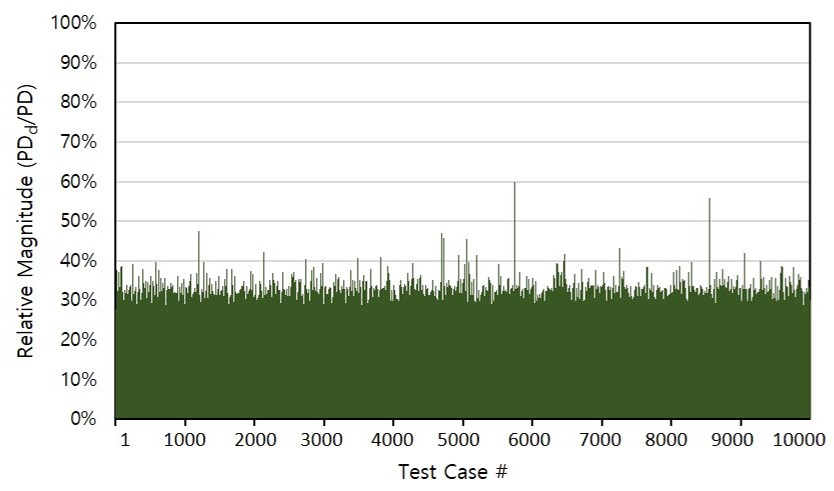Fig. 6. Relative magnitude of PDd over rigid penetration depth PD in 104 times of penetration resolution tests of randomly intersecting tetrahedron pairs. The average magnitude is 27.94%

# Contact

Ewha Graphics Lab
Department of Computer Science & Engineering, Ewha Womans University
52, Ewhayeodae-gil, Seodaemun-gu, Seoul, Korea, 03760
+82-2-3277-6798

Jisu Kim, cwyh5526@ewhain.net
Young J. Kim, kimy@ewha.ac.kr